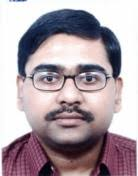# Measure Theoretic Probability 1

By Prof. Suprio Bhar   |   IIT Kanpur
Learners enrolled: 384
This course is aimed at the students who have already learnt about basic Probability distributions and Random variables, and are interested in learning the Mathematical formulation of Probability. We first discuss the modeling of sample space and events into a probability space and, Random variables as functions on such probability spaces. Then, using Measure Theoretic techniques, we discuss a theory of integration which unifies the formulas for the expectations of discrete and absolutely continuous Random variables. Towards the end, we look at various convergence theorems for expectations of Random variables and related inequalities. Students, who complete the course, should be able to apply these results towards advanced studies.

INTENDED AUDIENCE :Students who have already learnt about basic Probability distributions and Random variables, and are interested in learning the Mathematical formulation of Probability. PG/Ph.D students and senior UG students are welcome.
PRE-REQUISITES   : A good background of Real Analysis and Basis Probability Theory (covering Probability distributions and standard Random variables).
INDUSTRY SUPPORT : This is a course focused on the Mathematical foundations of Probability and not on applications. However, this course is useful prerequisite towards advanced courses such as Stochastic Calculus and Financial Mathematics. As such, most industries should recognize this course.
Summary
 Course Status : Completed Course Type : Elective Duration : 8 weeks Category : Mathematics Credit Points : 2 Level : Postgraduate Start Date : 20 Feb 2023 End Date : 14 Apr 2023 Enrollment Ends : 20 Feb 2023 Exam Registration Ends : 17 Mar 2023 Exam Date : 30 Apr 2023 IST

Note: This exam date is subjected to change based on seat availability. You can check final exam date on your hall ticket.

### Course layout

Week-1:Introduction to the course: A review of basic Probability and motivation towards the Mathematical formulation of Probability Theory; Fields and Sigma-fields of subsets of a non-empty set; Examples (emphasis on Borel sigma-fields on Euclidean spaces); Limits of sequences of events/sets, Monotone Class Theorem (Statement only)
Week-2:Measures and Measure spaces (Emphasis on Probabililty measures and Probability spaces); Examples and Properties
Week-3:Measurable functions (Emphasis on Random Variables); Examples:Properties (composition, algebraic properties, pointwise limits, measurability of components in higher dimensions); More examples using the above properties
Week-4:Caratheodery Extension Theorem (Emphasis on Uniqueness part, Existence part is statement only): Law/distribution of Random Variables; Distribution functions of Random Variables, properties
Week-5:Correspondence between Distribution functions and Probability measures on the real line; Extension of the above correspondence to higher dimensions (in brief); Lebesgue measure on the real line; Lebesgue measure on higher dimensions (in brief)
Week-6:Integration of measurable functions with respect to a measure (Emphasis on Expectation and Moments of Random Variables); Law/distribution of discrete Random variables, Example of measure theoretic integration: Expectation of discrete Random Variables; Convergence Theorems (Monotone Convergence Theorem, Fatou’s Lemma, Dominated Convergence Theorem), applications
Week-7:Convergence Theorems (Monotone Convergence Theorem, Fatou’s Lemma, Dominated Convergence Theorem), applications - Continued; Connection between Riemann and Lebesgue integration
Week-8:Radon-Nikodym Theorem (Statement only); Interpretation of the probabililty density function for absolutely continuous Random Variables as a Radom-Nikodym density, Law/distribution of absolutely continuous Random variables; Example of measure theoretic integration: Expectation of absolutely continuous Random Variables; inequalities involving Expectation and Moments of Random Variables; Conclusion of the course

### Books and references

1. Probability & Measure Theory (2nd Edition), Robert B. Ash, with contributions from Catherine A. Doleans-Dade. Elsevier.
2. A Course in Probability Theory (3rd Edition), Kai Lai Chung. Academic Press (Elsevier).
3. Probability and Measure (3rd Edition), Patrick Billingsley. Wiley.

### Instructor bio### Prof. Suprio Bhar

IIT Kanpur
Assistant Professor, Department of Mathematics and Statistics, Indian Institute of Technology Kanpur; Ph.D (Indian Statistical Institute, 2015); Research Interests: Stochastic PDEs

### Course certificate

The course is free to enroll and learn from. But if you want a certificate, you have to register and write the proctored exam conducted by us in person at any of the designated exam centres.
The exam is optional for a fee of Rs 1000/- (Rupees one thousand only).
Date and Time of Exams: 30 April 2023 Morning session 9am to 12 noon; Afternoon Session 2pm to 5pm.
Registration url: Announcements will be made when the registration form is open for registrations.
The online registration form has to be filled and the certification exam fee needs to be paid. More details will be made available when the exam registration form is published. If there are any changes, it will be mentioned then.
Please check the form for more details on the cities where the exams will be held, the conditions you agree to when you fill the form etc.

CRITERIA TO GET A CERTIFICATE

Average assignment score = 25% of average of best 6 assignments out of the total 8 assignments given in the course.
Exam score = 75% of the proctored certification exam score out of 100

Final score = Average assignment score + Exam score

YOU WILL BE ELIGIBLE FOR A CERTIFICATE ONLY IF AVERAGE ASSIGNMENT SCORE >=10/25 AND EXAM SCORE >= 30/75. If one of the 2 criteria is not met, you will not get the certificate even if the Final score >= 40/100.

Certificate will have your name, photograph and the score in the final exam with the breakup.It will have the logos of NPTEL and IIT Kanpur .It will be e-verifiable at nptel.ac.in/noc.

Only the e-certificate will be made available. Hard copies will not be dispatched.

Once again, thanks for your interest in our online courses and certification. Happy learning.

- NPTEL team